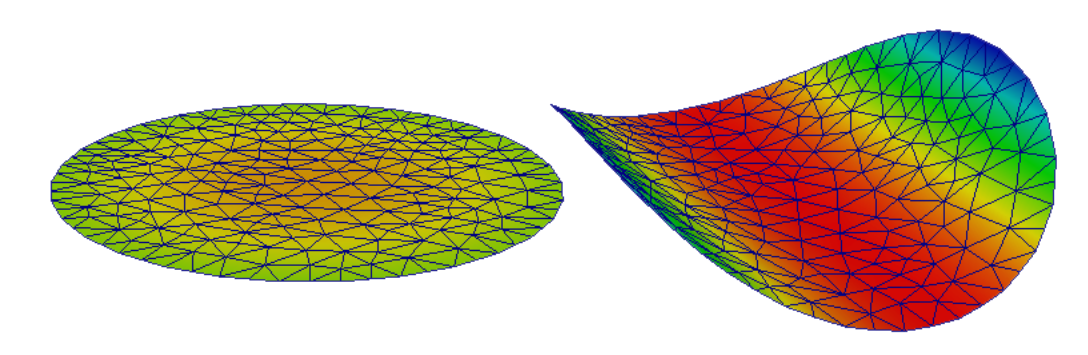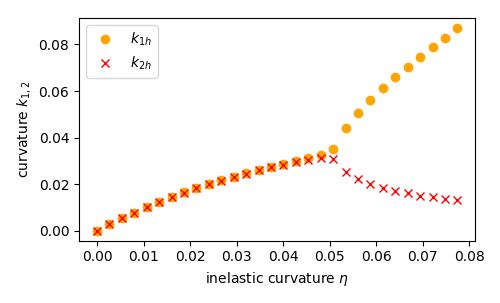# Buckling of a heated von-Kármán plate¶

This demo is implemented in the single Python file demo_von-karman-mansfield.py.

This demo program solves the von-Kármán equations on a circular plate with a lenticular cross section free on the boundary. The plate is heated, causing it to bifurcate. Bifurcation occurs with a striking shape transition: before the critical threshold the plate assumes a cup-shaped configurations (left); above it tends to a cylindrical shape (right). An analytical solution has been found by Mansfield, see .Pre-critical and post-critical plate configuration.

The standard von-Kármán theory gives rise to a fourth-order PDE which requires the transverse displacement field $$w$$ to be sought in the space $$H^2(\Omega)$$. We relax this requirement in the same manner as the Kirchoff-Love plate theory can be relaxed to the Reissner-Mindlin theory, resulting in seeking a transverse displacement field $$w$$ in $$H^1(\Omega)$$ and a rotation field $$\theta$$ in $$[H^2(\Omega)]^2$$. To alleviate the resulting shear- and membrane-locking issues we use Partial Selective Reduced Integration (PSRI), see .

To follow this demo you should know how to:

• Define a MixedElement and a FunctionSpace from it.
• Write variational forms using the Unified Form Language.
• Automatically derive Jabobian and residuals using derivative().
• Apply Dirichlet boundary conditions using DirichletBC and apply().
• Assemble forms using assemble().

This demo then illustrates how to:

• Define the Reissner-Mindlin-von-Kármán plate equations using UFL.
• Use the PSRI approach to simultaneously cure shear- and membrane-locking issues.

We start with importing the required modules, setting matplolib as plotting backend, and generically set the integration order to 4 to avoid the automatic setting of FEniCS which would lead to unreasonably high integration orders for complex forms.

from dolfin import *
from fenics_shells import *
import mshr
import numpy as np
import matplotlib.pyplot as plt



The mid-plane of the plate is a circular domain with radius $$a = 1$$. We generate a mesh of the domain using the package mshr:

a = 1.
ndiv = 8
domain_area = np.pi*a**2

centre = Point(0.,0.)

geom = mshr.Circle(centre, a)
mesh = mshr.generate_mesh(geom, ndiv)


The lenticular thinning of the plate can be modelled directly through the thickness parameter in the plate model:

t = 1E-2
ts = interpolate(Expression('t*(1.0 - (x*x + x*x)/(a*a))', t=t, a=a, degree=2), FunctionSpace(mesh, 'CG', 2))


We assume the plate is isotropic with constant material parameters, Young’s modulus $$E$$, Poisson’s ratio $$\nu$$; shear correction factor $$\kappa$$:

E = Constant(1.0)
nu = Constant(0.3)
kappa = Constant(5.0/6.0)


Then, we compute the (scaled) membrane, $$A/t^3$$, bending, $$D/t^3$$, and shear, $$S/t^3$$, plate elastic stiffnesses:

A = (E*ts/t**3/(1. - nu**2))*as_tensor([[1., nu, 0.],[nu, 1., 0.],[0., 0., (1. - nu)/2]])
D = (E*ts**3/t**3/(12.*(1. - nu**2)))*as_tensor([[1., nu, 0.],[nu, 1., 0.],[0., 0., (1. - nu)/2]])
S = E*kappa*ts/t**3/(2*(1. + nu))


We use a $$CG_2$$ element for the in-plane and transverse displacements $$u$$ and $$w$$, and the enriched element $$[CG_1 + B_3]$$ for the rotations $$\theta$$. We collect the variables in the state vector $$q =(u,w,\theta)$$:

P1 = FiniteElement("Lagrange", triangle, degree = 1)
P2 = FiniteElement("Lagrange", triangle, degree = 2)
bubble = FiniteElement("B", triangle, degree = 3)
enriched = P1 + bubble

element = MixedElement([VectorElement(P2, dim=2), P2, VectorElement(enriched, dim=2)])

Q = FunctionSpace(mesh, element)


Then, we define Function, TrialFunction and TestFunction objects to express the variational forms and we split them into each individual component function:

q_, q, q_t = Function(Q), TrialFunction(Q), TestFunction(Q)
v_, w_, theta_ = split(q_)


The membrane strain tensor $$e$$ for the von-Kármán plate takes into account the nonlinear contribution of the transverse displacement in the approximate form:

$e(v, w) = \mathrm{sym}\nabla v + \frac{\nabla w \otimes \nabla w}{2}$

which can be expressed in UFL as:

e = sym(grad(v_)) + 0.5*outer(grad(w_), grad(w_))


The membrane energy density $$\psi_m$$ is a quadratic function of the membrane strain tensor $$e$$. For convenience, we use our function strain_to_voigt() to express $$e$$ in Voigt notation $$e_V = \{e_1, e_2, 2 e_{12}\}$$:

ev = strain_to_voigt(e)
psi_m = 0.5*dot(A*ev, ev)


The bending strain tensor $$k$$ and shear strain vector $$\gamma$$ are identical to the standard Reissner-Mindlin model. The shear energy density $$\psi_s$$ is a quadratic function of the shear strain vector:

gamma = grad(w_) - theta_
psi_s = 0.5*dot(S*gamma, gamma)


The bending energy density $$\psi_b$$ is a quadratic function of the bending strain tensor. Here, the temperature profile on the plate is not modelled directly. Instead, it gives rise to an inelastic (initial) bending strain tensor $$k_T$$ which can be incoporated directly in the Lagrangian:

k_T = as_tensor(Expression((("c/imp","0.0"),("0.0","c*imp")), c=1., imp=.999,  degree=0))
kv = strain_to_voigt(k)
psi_b = 0.5*dot(D*kv, kv)


Note

The standard von-Kármán model can be recovered by substituting in the Kirchoff constraint $$\theta = \nabla w$$.

Shear- and membrane-locking are treated using the partial reduced selective integration proposed by Arnold and Brezzi, see . In this approach shear and membrane energy are splitted as a sum of two contributions weighted by a factor $$\alpha$$. One of the two contributions is integrated with a reduced integration. We use $$2\times 2$$-points Gauss integration for a portion $$1-\alpha$$ of the energy, whilst the rest is integrated with a $$4\times 4$$ scheme. We adopt an optimized weighting factor $$\alpha=(t/h)^2$$, where $$h$$ is the mesh size.:

dx_h = dx(metadata={'quadrature_degree': 2})
h = CellDiameter(mesh)
alpha = project(t**2/h**2, FunctionSpace(mesh,'DG',0))

Pi_PSRI = psi_b*dx + alpha*psi_m*dx + alpha*psi_s*dx + (1.0 - alpha)*psi_s*dx_h + (1.0 - alpha)*psi_m*dx_h


Then, we compute the total elastic energy and its first and second derivatives:

Pi = Pi_PSRI
dPi = derivative(Pi, q_, q_t)
J = derivative(dPi, q_, q)


This problem is a pure Neumann problem. This leads to a nullspace in the solution. To remove this nullspace, we fix all the variables in the central point of the plate and the displacements in the $$x_0$$ and $$x_1$$ direction at $$(0, a)$$ and $$(a, 0)$$, respectively:

zero_v1 = project(Constant((0.)), Q.sub(0).sub(0).collapse())
zero_v2 = project(Constant((0.)), Q.sub(0).sub(1).collapse())
zero = project(Constant((0.,0.,0.,0.,0.)), Q)

bc = DirichletBC(Q, zero, "near(x, 0.) and near(x, 0.)", method="pointwise")
bc_v1 = DirichletBC(Q.sub(0).sub(0), zero_v1, "near(x, 0.) and near(x, 1.)", method="pointwise")
bc_v2 = DirichletBC(Q.sub(0).sub(1), zero_v2, "near(x, 1.) and near(x, 0.)", method="pointwise")
bcs = [bc, bc_v1, bc_v2]


Then, we define the nonlinear variational problem and the solver settings:

init = Function(Q)
q_.assign(init)
problem = NonlinearVariationalProblem(dPi, q_, bcs, J = J)
solver = NonlinearVariationalSolver(problem)
solver.parameters["newton_solver"]["absolute_tolerance"] = 1E-8


Finally, we choose the continuation steps (the critical loading c_cr is taken from the Mansfield analytical solution ):

c_cr = 0.0516
cs = np.linspace(0.0, 1.5*c_cr, 30)


and we solve as usual:

defplots_dir = "output/3dplots-psri/"
file = File(defplots_dir + "sol.pvd")

ls_kx = []
ls_ky = []
ls_kxy = []
ls_kT = []

for count, i in enumerate(cs):
k_T.c = i
solver.solve()
v_h, w_h, theta_h = q_.split(deepcopy=True)


To visualise the solution we assemble the bending strain tensor:

K_h = project(sym(grad(theta_h)), TensorFunctionSpace(mesh, 'DG', 0))


we compute the average bending strain:

Kxx = assemble(K_h[0,0]*dx)/domain_area
Kyy = assemble(K_h[1,1]*dx)/domain_area
Kxy = assemble(K_h[0,1]*dx)/domain_area

ls_kT.append(i)
ls_kx.append(Kxx)
ls_ky.append(Kyy)
ls_kxy.append(Kxy)


and output the results at each continuation step:

v1_h, v2_h = v_h.split()
u_h = as_vector([v1_h, v2_h, w_h])
u_h_pro = project(u_h, VectorFunctionSpace(mesh, 'CG', 1, dim=3))
u_h_pro.rename("q_","q_")
file << u_h_pro


Finally, we plot the average curvatures as a function of the inelastic curvature:

fig = plt.figure(figsize=(5.0, 5.0/1.648))
plt.plot(ls_kT, ls_kx, "o", color='orange', label=r"$k_{1h}$")
plt.plot(ls_kT, ls_ky, "x", color='red', label=r"$k_{2h}$")
plt.xlabel(r"inelastic curvature $\eta$")
plt.ylabel(r"curvature $k_{1,2}$")
plt.legend()
plt.tight_layout()
plt.savefig("psri-%s.png"%ndiv)Comparison with the analytical solution.

## Unit testing¶

import pytest
@pytest.mark.skip
def test_close():
pass